Next: Expansion of Up: Utilities Previous: Derivative of a polynomial   Contents

Euclidian division

The procedure:

void ALIAS_Euclidian_Division(int Degree,INTERVAL_VECTOR &Coeff1,
INTERVAL_VECTOR &Coeff2,INTERVAL &Residu,INTERVAL &a)
computes the division of the polynomial P with interval coefficients Coeff1 by x-a and returns the polynomial P1 with coefficients Coeff2 such that P=(x-a)P1+Residu

Another procedure that perform the same process if a is a double is

void Divide_Single(int Degree,INTERVAL_VECTOR &Coeff1,double a,
INTERVAL_VECTOR &Coeff2, INTERVAL &Residu)
For dividing a polynomial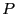by the product of polynomials of type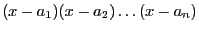so that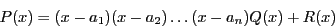and evaluating the polynomial under this form for some interval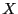for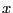you may use

INTERVAL Divide_Evaluate_Multiple(int Degree,INTERVAL_VECTOR &Coeff1,
int n,double *a,INTERVAL_VECTOR &X)
where
• n: the number of terms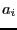• a: the value of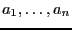The interval returned by this procedure is the the interval evaluation offorhaving the the interval value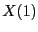.

For the Euclidian division by an arbitrary polynomial you may use

int Divide_Polynom(int Degree,INTERVAL_VECTOR &Coeff,int DegreeDivisor,
INTERVAL_VECTOR &CoeffDivisor,
INTERVAL_VECTOR &CoeffQuo, INTERVAL_VECTOR &CoeffRem)
The polynomialof degree Degree with cofficients Coeff will be divided by the polynomial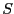of degree DegreeDivisor with coefficients CoeffDivisor so that the coefficient will be a polynomial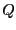of degree Degree-DegreeDivisor with coefficients CoeffQuo with a reminder which is a polynomial of degree DegreeDivisor and coefficients CoeffRem. This procedure returns 0 if the division is not possible (the interval coefficient of the leading term ofincludes 0) or if the degree ofis greater than the degree of. If the division has been performed this procedure will return 1.

Note that Divide_Polynom and Divide_Evaluate_Multiple may be used to perform the deflation of an univariate polynomial as soon as approximate roots for the polynomial have been found. This may be useful to decrease the computation time for solving a polynomial or for numerically instable polynomial such as the Wilkinson polynomial. A combination of Rouche filtering and of deflation allows to solve Wilkinson polynomial of order 18, while the general solving procedure will fail starting at order 13.

A special Euclidian division is the division of a polynomialby its derivative. This can be done with

void Quotient_UP_Derivative(int Degree,VECTOR &Coeff,VECTOR &CoeffD,VECTOR &Quo,
VECTOR &Rem)
wherehas coefficients Coeff, its derivative CoeffD and the coefficient of the division is Quo (the quotient is a polynomial of degree 1) while the coefficients of the remainder are stored in RemNext: Expansion of Up: Utilities Previous: Derivative of a polynomial   Contents
Jean-Pierre Merlet 2012-12-20